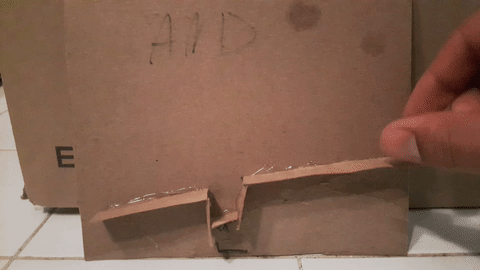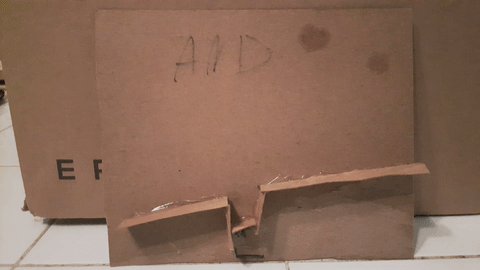# What is a computer?¶

Given some input, a computer automates a series of logical steps to produce some output.

Very abstractly, suppose $$A$$ is either TRUE or FALSE and $$B$$ is either TRUE or FALSE and we want to know if $$A$$ is TRUE and $$B$$ is TRUE. A computer can take $$A$$ and $$B$$ as inputs and output $$C$$, which is TRUE if $$A$$ and $$B$$ are TRUE and FALSE otherwise. The logical step in this case is just determining if $$A$$ and $$B$$ are TRUE.

More concretely, this person implemented this computer with cardboard and marbles. The “computer” is a track with a pit in the middle. The inputs are whether you roll no marbles, one marble or two marbles down the track. If you roll no marbles, then $$A$$ and $$B$$ are FALSE. If you roll one marble, then $$A$$ is TRUE and $$B$$ is FALSE. If you roll two marbles, then $$A$$ and $$B$$ are TRUE. The output is whether we observe a marble rolling off the end of the track. If we observe a marble rolling off the end of the track, that means $$A$$ and $$B$$ must be TRUE.This is a pretty dumb and useless computer, but imagine having 1000 inputs and a very complicated series of logical steps (e.g. if the first 10 inputs are true, but the last input is false or if the 15th input is true, then the first output is true but the second one is false, …). Then, it might be useful not to have to go through the logical steps for every new set of 1000 inputs that you have, but to just be able to throw a bunch of marbles into a gigantic cardboard maze and have it give you the answer. Like this calculator: